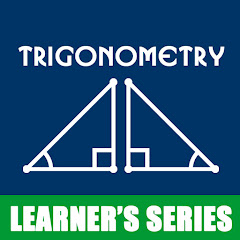# Trigonometry Mathematics

In-app purchases
4.2
1.14K reviews
100K+Everyone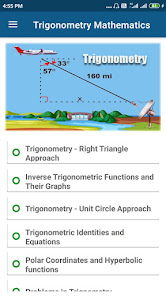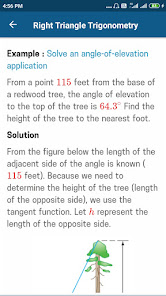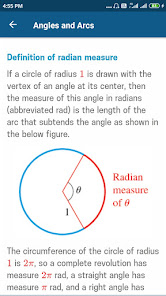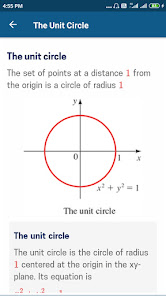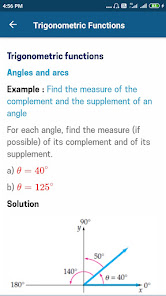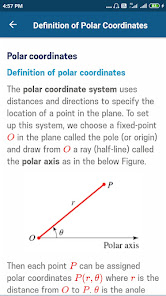Application designed for beginners to learn the basics of trigonometry. This explains completely its mathematical and geometrical interpretation and physical significances. Deals all about trigonometric formulas and identities. Very helpful tutorials for students to solve mathematical problems under the category of heights and distances…try out now…

✔ Introduction Trigonometry
✔ Angle (Trigonometry)
✔ Quadrant Angle (Trigonometry)
✔ Co-terminal angles
✔ Trig functions of large and negative angles
✔ Reference angle
✔ Trigonometry functions - introduction
✔ SOH CAH TOA - trigonometry
✔ Inverse trigonometry functions
✔ Trig functions of large and negative angles
✔ Sine (sin) function - Trigonometry
✔ Arcsin
✔ Graph of the sine (sin) function - Trigonometry
✔ Sine waves - Trigonometry
✔ Cosine (cos) function - Trigonometry
✔ Arccos
✔ Graph of the cosine (cos) function - Trigonometry
✔ Tangent (tan) function - Trigonometry
✔ Arctan
✔ Graph of the tangent (tan) function - Trigonometry
✔ Cotangent
✔ Secant (sec) - Trigonometry function
✔ Cosecant (cosec) - Trigonometry function
Updated on
Nov 19, 2020

## Data safety

Developers can show information here about how their app collects and uses your data. Learn more about data safetyNo information available
4.2
1.14K reviews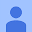October 27, 2019
Amazing app but it would be nice if you can make diagrams zoomable so we can see all images more clearly.
200 people found this review helpful
Did you find this helpful?
Learner's Series
November 30, 2020
Dear user , Thank you very much for sharing your valuable & useful feedback. Application updated now, zooming provision has been enabled. Latest version available now.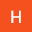Husen Waritu
June 16, 2022
Good service app But how can you obtain more information about the application .e.g adjoint of matrix and the graph of trigonometry function
8 people found this review helpful
Did you find this helpful?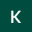Keneuwe Nkoko
August 2, 2021
Very helpful for me, very useful and it's perfect for exam preparations
53 people found this review helpful
Did you find this helpful?